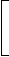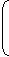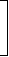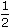# Aptitude - True Discount

### Exercise :: True Discount - General Questions

11.

Rs. 20 is the true discount on Rs. 260 due after a certain time. What will be the true discount on the same sum due after half of the former time, the rate of interest being the same?

 A. Rs. 10 B. Rs. 10.40 C. Rs. 15.20 D. Rs. 13

Explanation:

S.I. on Rs. (260 - 20) for a given time = Rs. 20.

S.I. on Rs. 240 for half the time = Rs. 10.

T.D. on Rs. 250 = Rs. 10.T.D. on Rs. 260 = Rs.10 x 260= Rs. 10.40 250

12.

The interest on Rs. 750 for 2 years is the same as the true discount on Rs. 960 due 2 years hence. If the rate of interest is the same in both cases, it is:

A. 12%
B. 14%
C. 15%
D.
 16 2 % 3

Explanation:

S.I. on Rs. 750 = T.D. on Rs. 960.

This means P.W. of Rs. 960 due 2 years hence is Rs. 750.T.D. = Rs. (960 - 750) = Rs. 210.

Thus, S.I. on R.s 750 for 2 years is Rs. 210.Rate =100 x 210% = 14% 750 x 2

13.

The simple interest and the true discount on a certain sum for a given time and at a given rate are Rs. 85 and Rs. 80 respectively. The sum is:

 A. Rs. 1800 B. Rs. 1450 C. Rs. 1360 D. Rs. 6800

Explanation:

 Sum = S.I. x T.D. = 85 x 80 = Rs. 1360. (S.I.) - (T.D.) (85 - 80)

14.

The present worth of Rs. 1404 due in two equal half-yearly installments at 8% per annum simple interest is:

 A. Rs. 1325 B. Rs. 1300 C. Rs. 1350 D. Rs. 1500

Explanation:

Required sum = P.W. of Rs. 702 due 6 months + P.W. of Rs. 702 due 1 year hence
= Rs.100 x 702+100 x 702100 + (8 x 1)100 + 8 x= Rs. (675 + 650)
= Rs. 1325.

15.

If the true discount on s sum due 2 years hence at 14% per annum be Rs. 168, the sum due is:

 A. Rs. 768 B. Rs. 968 C. Rs. 1960 D. Rs. 2400

Explanation:

 P.W. = 100 x T.D. = 100 x 168 = 600. R x T 14 x 2Sum = (P.W. + T.D.) = Rs. (600 + 168) = Rs. 768.